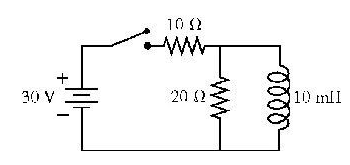# Problem: The switch in the figure has been open for a long time. It is closed at t = 0 s.What is the current through the 20 ohm resistor immediately after the switch is closed?

###### FREE Expert Solution

For resistors in series:

Ohm's law:

$\overline{){\mathbf{V}}{\mathbf{=}}{\mathbf{i}}{\mathbf{R}}}$

The inductor acts as a path of infinite resistance (open circuit) immediately after the switch is closed.

82% (320 ratings)###### Problem Details

The switch in the figure has been open for a long time. It is closed at t = 0 s.What is the current through the 20 ohm resistor immediately after the switch is closed?

Frequently Asked Questions

What scientific concept do you need to know in order to solve this problem?

Our tutors have indicated that to solve this problem you will need to apply the LR Circuits concept. You can view video lessons to learn LR Circuits. Or if you need more LR Circuits practice, you can also practice LR Circuits practice problems.